Question

# Do each question separately and show step by step Two spherical objects are separated by a...

Do each question separately and show step by step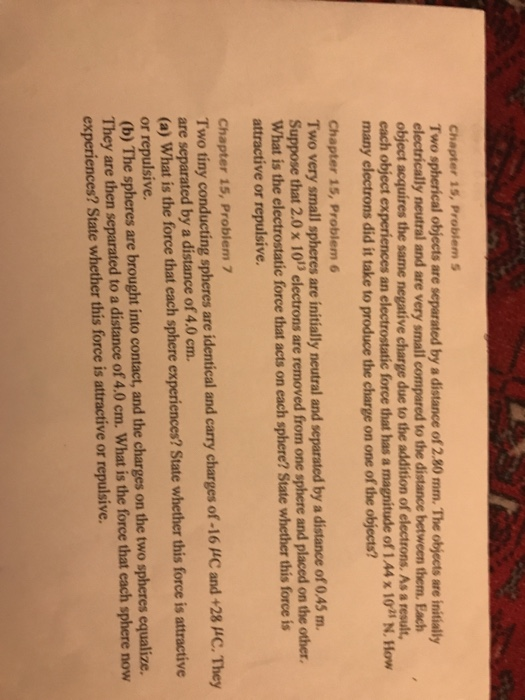Two spherical objects are separated by a distance of 2.80 mm. The objects are initially electrically neutral and are very small compared to the distance between them. Each object acquires the same negative charge due to the addition of electrons, As a result, each object experiences an electrostatic force that has a magnitude of 1.44 times 10^-25 N. How many electrons did it take to produce the charge on one of the objects? Two very small spheres are initially neutral and separated by a distance of 0.45 m. Suppose that 2.0 times 10^13 electrons are removed from one sphere and placed on the other, What is the electrostatic force that acts on each sphere? State whether this force is attractive or repulsive. Two tiny conducting spheres are identical and carry charges of -16 mu C and +28 mu C. They are separated by a distance of 4.0 cm. (a) What is the force that each sphere experiences? State whether this force is attractive or repulsive (b) The spheres are brought into contact, and the charges on the two spheres equalize. they are then separated to a distance of 4.0 cm. What is the force that each sphere now experiences? State whether this force is attractive or repulsive.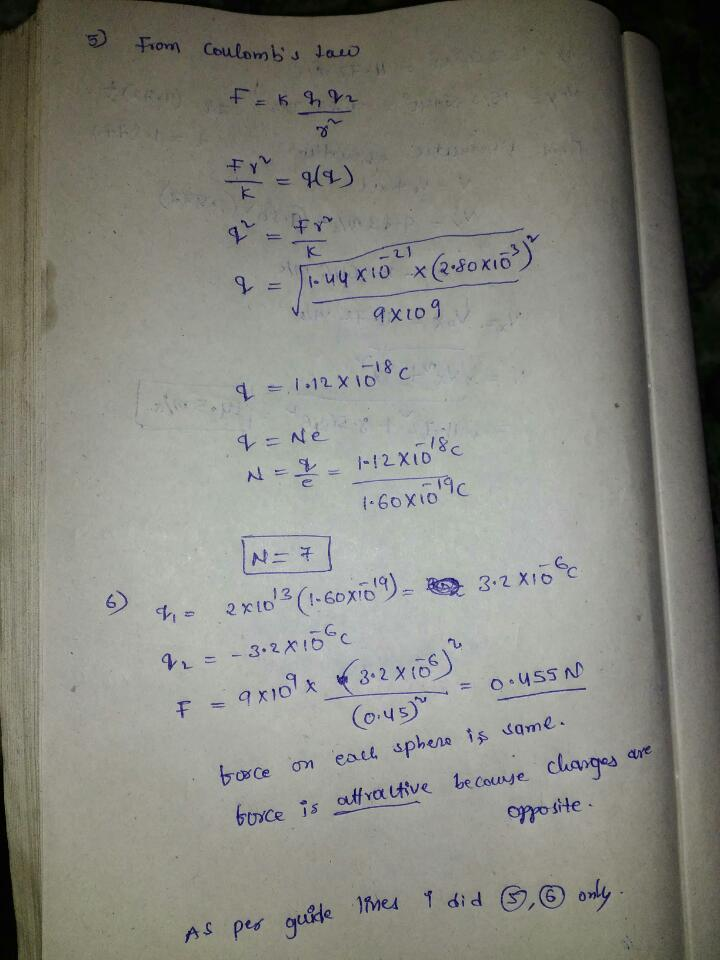#### Earn Coins

Coins can be redeemed for fabulous gifts.

Similar Homework Help Questions
• ### CJ10 18 P.009 Two spherical objects are separated by a distance that is 5.40 x 103...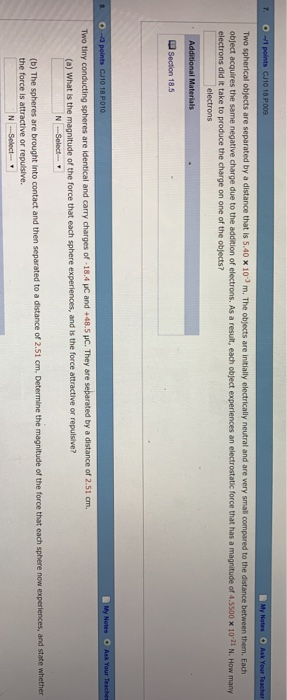CJ10 18 P.009 Two spherical objects are separated by a distance that is 5.40 x 103 m. The objects are initially electrically neutral and are very small o ect acquires the same negative charge due to the addition of electrons. As a result, each object experiences an electrostatic force that has a magnitude of 4 SS00 x 10-21 N How many electrons did it take to produce the charge on one of the objects? electrons CJ10 18 P010 Two tinyc...

• ### Two spherical objects are separated by a distance that is 9.00 10-3 m. The objects are...

Two spherical objects are separated by a distance that is 9.00 10-3 m. The objects are initially electrically neutral and are very small compared to the distance between them. Each object acquires the same negative charge due to the addition of electrons. As a result, each object experiences an electrostatic force that has a magnitude of 1.1375 10-21 N. How many electrons did it take to produce the charge on one of the objects? electrons?

• ### Two spherical objects are separated by a distance of 1.25 × 10-3 m. The objects are...

Two spherical objects are separated by a distance of 1.25 × 10-3 m. The objects are initially electrically neutral and are very small compared to the distance between them. Each object acquires the same negative charge due to the addition of electrons. As a result, each object experiences an electrostatic force that has a magnitude of 1.78 × 10-20 N. How many electrons did it take to produce the charge on one of the objects?

• ### Two spherical objects are separated by a distance of 1.84 × 10-3 m. The objects are...

Two spherical objects are separated by a distance of 1.84 × 10-3 m. The objects are initially electrically neutral and are very small compared to the distance between them. Each object acquires the same negative charge due to the addition of electrons. As a result, each object experiences an electrostatic force that has a magnitude of 8.23 × 10-21 N. How many electrons did it take to produce the charge on one of the objects?

• ### Two spherical objects are separated by a distance of 2.04 × 10-3 m. The objects are...

Two spherical objects are separated by a distance of 2.04 × 10-3 m. The objects are initially electrically neutral and are very small compared to the distance between them. Each object acquires the same negative charge due to the addition of electrons. As a result, each object experiences an electrostatic force that has a magnitude of 1.08 × 10-20 N. How many electrons did it take to produce the charge on one of the objects?​

• ### Two spherical objects are separated by a distance of 1.47 × 10-3 m. The objects are...

Two spherical objects are separated by a distance of 1.47 × 10-3 m. The objects are initially electrically neutral and are very small compared to the distance between them. Each object acquires the same negative charge due to the addition of electrons. As a result, each object experiences an electrostatic force that has a magnitude of 1.53 × 10-20 N. How many electrons did it take to produce the charge on one of the objects?

• ### Two spherical objects are separated by a distance of 1.07 x 102m. The objects are initially...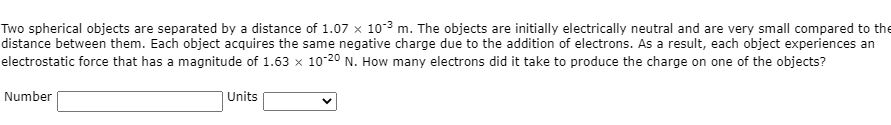Two spherical objects are separated by a distance of 1.07 x 102m. The objects are initially electrically neutral and are very small compared to the distance between them. Each object acquires the same negative charge due to the addition of electrons. As a result, each object experiences an electrostatic force that has a magnitude of 1.63 x 10-20 N. How many electrons did it take to produce the charge on one of the objects? Number Units

• ### Two spherical objects are separated by a distance of 1.01 × 10-3 m. The objects are initially electrically neutral and are very small compared to the distance between them. Each object acquires the sa...

Two spherical objects are separated by a distance of 1.01 × 10-3 m. The objects are initially electrically neutral and are very small compared to the distance between them. Each object acquires the same negative charge due to the addition of electrons. As a result, each object experiences an electrostatic force that has a magnitude of 1.83 × 10-20 N. How many electrons did it take to produce the charge on one of the objects?

• ### Twe spherical objects are separated by a distance of 2.01 x 10 m. The objects are...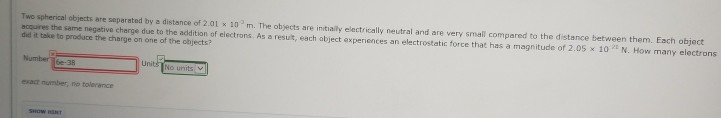Twe spherical objects are separated by a distance of 2.01 x 10 m. The objects are initialy elactrically neutral and are very small compared to the distance between them. Each object acquires the same negetive charge due to the addition of electrons. As a resut, each abject experiences an electrostatic force that has a magnitude of 2.05 x 10 N. How many electrons did it take to produce the charge on one of the objects? exat number, no tolerance

• ### Two tiny conducting spheres are identical and carry charges of -21.6 µC and +45.5 µC. They...

Two tiny conducting spheres are identical and carry charges of -21.6 µC and +45.5 µC. They are separated by a distance of 2.37 cm. (a) What is the magnitude of the force that each sphere experiences, and is the force attractive or repulsive? (b) The spheres are brought into contact and then separated to a distance of 2.37 cm. Determine the magnitude of the force that each sphere now experiences, and state whether the force is attractive or repulsive.